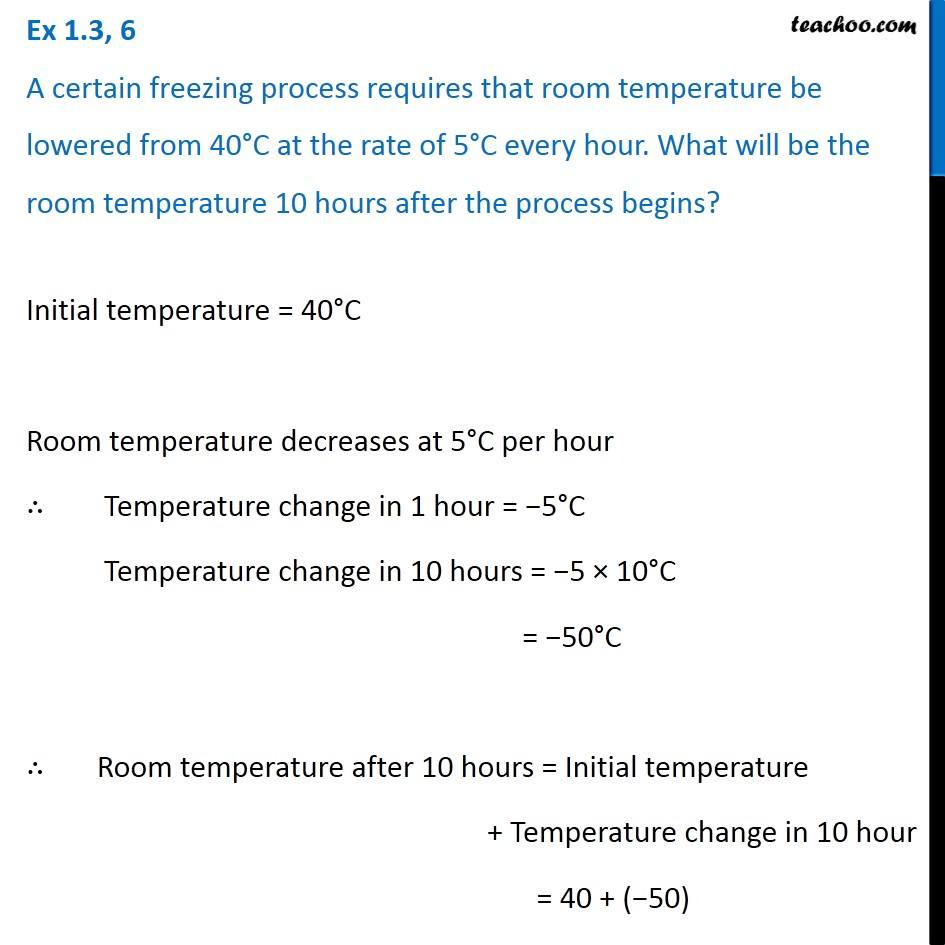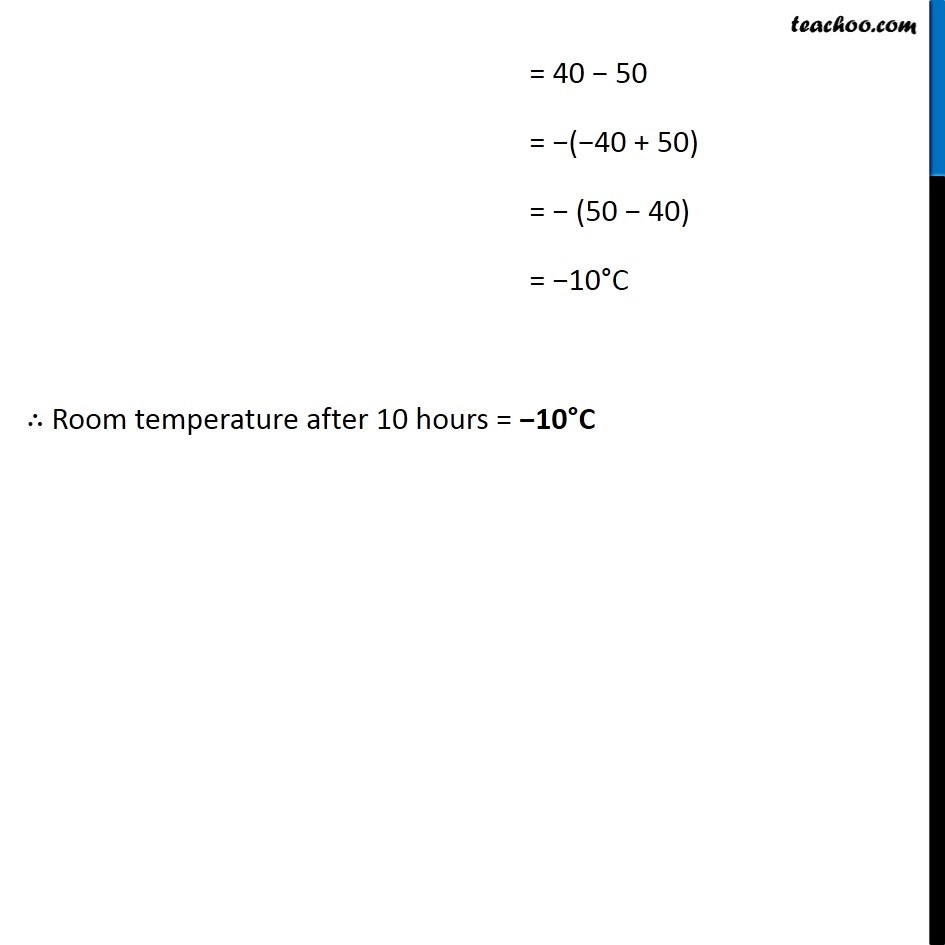Statement Questions - Multiplication

Chapter 1 Class 7 Integers
Concept wiseLearn in your speed, with individual attention - Teachoo Maths 1-on-1 Class

### Transcript

Question 2 A certain freezing process requires that room temperature be lowered from 40 C at the rate of 5 C every hour. What will be the room temperature 10 hours after the process begins? Initial temperature = 40 C Room temperature decreases at 5 C per hour Temperature change in 1 hour = 5 C Temperature change in 10 hours = 5 10 C = 50 C Room temperature after 10 hours = Initial temperature + Temperature change in 10 hour = 40 + ( 50) = 40 50 = ( 40 + 50) = (50 40) = 10 C Room temperature after 10 hours = 10 C